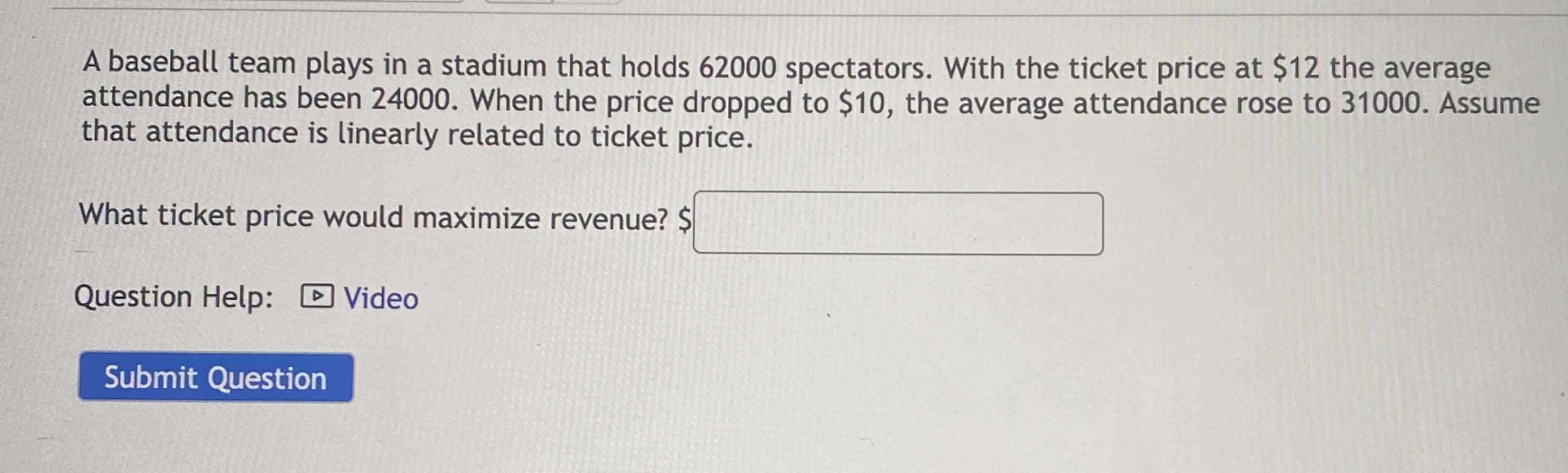### ¿Todavía tienes preguntas de matemáticas?

Pregunte a nuestros tutores expertos
Algebra
PreguntaA baseball team plays in a stadium that holds $$62000$$ spectators. With the ticket price at $$\ 12$$ the average attendance has been $$24000$$ . When the price dropped to $$\ 10$$ , the average attendance rose to $$31000$$ . Assume that attendance is linearly related to ticket price. What ticket price would maximize revenue?